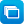# Mathematics / Year 8 / Statistics and Probability / Chance

Curriculum content descriptions

Describe events using language of 'at least', exclusive 'or' (A or B but not both), inclusive 'or' (A or B or both) and 'and'. (ACMSP205)

Elaborations
• posing 'and', 'or' and 'not' probability questions about objects or people
General capabilities
• Literacy Literacy
• Numeracy Numeracy
ScOT terms

Probability,  Boolean operators

## Refine results by

Year level 7-8
Resource type
Learning area Mathematics

## Refine by topic

Related topic### Snakes and spinners: assessment

Assess your understanding of chance in a practical situation. Use a spinner to move a game character along a path. Determine the least number of spins to finish a game. Determine the maximum number of spins to finish a game. Calculate the average number of steps taken per spin. Compare two spinners to decide which will ...### TIMES Module 13: Statistics and Probability: chance, year 8 - teacher guide

This is a 15-page guide for teachers. It continues the development of probability. A careful consideration of outcomes and equally likely outcomes is undertaken. In year 8, students see that these are a special case of finding probabilities of events by summing probabilities of the disjoint (or mutually exclusive) outcomes ...### Exploring probability

Look at the probability of a snowboarder choosing any one of six ski runs down a mountain. Compare expected probabilities with experimental results. Examine outcomes illustrated in graphs and probabilities expressed as fractions and percentages. Notice there is likely to be a greater variation between expected and actual ...### Introducing chance - middle years

This collection of 19 digital curriculum resources is organised into three categories: random sampling and predicting outcomes; random sampling from a population; and predictions and outcomes. It contains selections from four interactive learning object series: Vile vendor; Foul food; Random or not; and Dice duels. In these ...### Thumb wrestling tournament

In this introductory activity students use a simple thumb-wrestling tournament to analyse a series of matches in which there can only be one victor. Students work in small groups to explore different ways of mapping out the events of a tournament, introducing the concept of constructing sample spaces and tree diagrams as ...### Games of chance - unit of work

This unit provides students with a real life context to investigate chance by exploring and creating games of chance.### Red balls: assessment

Assess your ability to find the location of two red balls hidden under two of eight possible cups. Select combinations of two cups at a time. You will have six tries to guess the location of the balls. Use the feedback provided to help you. (It will indicate whether there are one, two or no balls in the cup combination you chose.)### Two-way tables

This is a four-page HTML resource about two-way tables. It contains three videos and four questions, three of which are interactive. The resource discusses and explains two-way tables and provides questions involving two-way tables to reinforce students' understanding.### Language of chance - unit of work

This unit provides students with a real life context to investigate the language of chance.### Take a chance - unit of work

This unit provides students with a real life context to investigate the language of chance and how events may be manipulated to alter the chance of something happening. It also introduces the use of fractions, decimals and percentages when looking at probability.